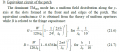# How to calculate input impedance (edge) for this microstrip Patch antenna?

#### KaiL

Joined Aug 30, 2014
69
1234

Last edited:

#### MrAl

Joined Jun 17, 2014
6,488
Hi,

Perhaps you can get the formula from the first website and compare it to the other formula and see if they are similar. One possibility is that the web site did not set up the formula right so you could try that.

#### KaiL

Joined Aug 30, 2014
69
Hi,

Perhaps you can get the formula from the first website and compare it to the other formula and see if they are similar. One possibility is that the web site did not set up the formula right so you could try that.
Actually I am not sure if the formula is correct or wrong since this research article (Page 4) uses the formula from the website - microstrip calculator , to find the Rin|y=0
http://www.ijafrc.org/Volume2/issue1/1.pdf

Rin | y=0 = 243 ohm (obtained using the microstrip calculator) and it uses this value to find yo. However , I am not sure how the yo is found.

Given the formula
50 = 243 cos^2(pi*A/L) whereby A = yo
50 = 243 cos^2(pi*A/L)
Divide by 243:
cos^2(pi*A/L) = 50 / 243
Take the square root:
cos (pi*A/L) = (5 √2) / 243
Take the arccos:
pi*A/L = arccos [(5 √2) / 243]
divide by pi:
A/L = arccos [(5 √2) / 243] / pi
Evaluate the right-hand side:
A/L = 0.490736
Multiply by L:
A = 0.490736 L
Substitute the value for L:
A = 0.490736 (28.30) = 13.9

A is around 13.9 which is different from the research article of y0 = 8.1

The research article shows that y0 = 8.1mm but my calculated value is 13.9

#### MrAl

Joined Jun 17, 2014
6,488
Hi,

You mean they already got the formula from the website?

#### Tesla23

Joined May 10, 2009
374
The formula for G1 in that paper doesn't look right. I think the original reference for this is the paper by Carver "Practical analytical techniques for the microstrip antenna" which you can find here www.dtic.mil/dtic/tr/fulltext/u2/a104404.pdf - starting page 7-1, G is given by eqn 12.

A more modern treatment is here: http://www.ece.mcmaster.ca/faculty/nikolova/antenna_dload/current_lectures/L20_Mstrip1.pdf
these are lecture notes written by an IEEE Fellow, so have a reasonable chance of being correct.using (21.6) and only the first term, gives 303 ohms at each end, or 152 ohms total. This is an approximation, it doesn't include G12, or the correction to G1 to account for the patch width. If you include these you might get 144 ohms, I don't know what they are claiming to calculate.

The document you refer to doesn't appear to have been peer reviewed, so hasn't had much checking.

#### MrAl

Joined Jun 17, 2014
6,488
Hi,

That's one of the reasons i hate answering questions like this, because there are various estimates and sometimes they dont even mention that it is just an estimate and not a solid total physics based result.